Chapter 1: Algebra Review

# 1.1 Integers

The ability to work comfortably with negative numbers is essential to success in algebra. For this reason, a quick review of adding, subtracting, multiplying, and dividing integers is necessary. Integers are all the positive whole numbers, all the negative whole numbers, and zero. As this is intended to be a review of integers, descriptions and examples will not be as detailed as in a normal lesson.

When adding integers, there are two cases to consider. The first is when the signs match—that is, the two integers are both positive or both negative.

If the signs match, add the numbers together and retain the sign.

Example 1.1.1

Add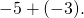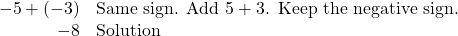Example 1.1.2

Add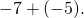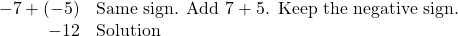The second case is when the signs don’t match, and there is one positive and one negative number. Subtract the numbers (as if they were all positive), then use the sign from the number with the greatest absolute value. This means that, if the number with the greater absolute value is positive, the answer is positive. If it is negative, the answer is negative.

Example 1.1.3

Add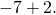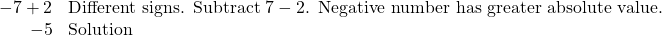Example 1.1.4

Add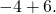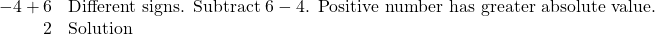Example 1.1.5

Add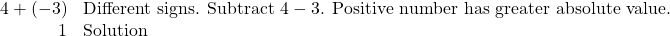Example 1.1.6

Add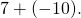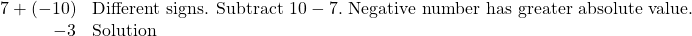For subtraction of negatives, change the problem to an addition problem, which is then solved using the above methods. The way to change a subtraction problem to an addition problem is by adding the opposite of the number after the subtraction sign to the number before the subtraction sign. Often, this method is referred to as “adding the opposite.”

Example 1.1.7

Subtract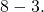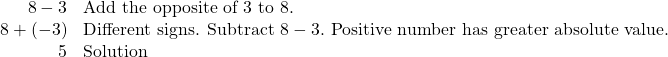Example 1.1.8

Subtract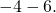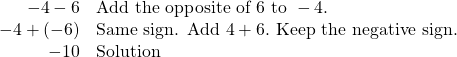Example 1.1.9

Subtract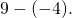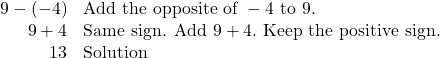Example 1.1.10

Subtract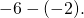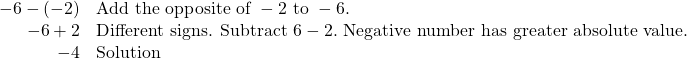Multiplication and division of integers both work in a very similar pattern. The short description of the process is to multiply and divide like normal. If the signs match (numbers are both positive or both negative), the answer is positive. If the signs don’t match (one positive and one negative), then the answer is negative.

Example 1.1.11

Multiply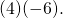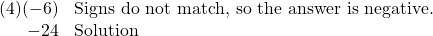Example 1.1.12

Divide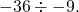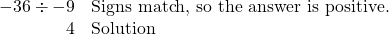Example 1.1.13

Multiply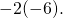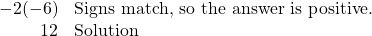Example 1.1.14

Divide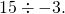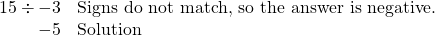Key Takeaways: A few things to be careful of when working with integers.

Be sure not to confuse a problem like −3 − 8 with −3(−8).

• The −3 − 8 problem is subtraction because the subtraction sign separates the −3 from what comes after it.
• The −3(−8) is a multiplication problem because there is nothing between the −3 and the parenthesis. If there is no operation written in between the parts, then you assume that you are multiplying.

Be careful not to mix the pattern for adding and subtracting integers with the pattern for multiplying and dividing integers. They can look very similar. For example:

• If the two numbers in an addition problem are negative, then keep the negative sign, such as in −3 + (−7)  = −10.
• If the signs of the two numbers in a multiplication problem match, the answer is positive, such as in (−3)(−7)  = 21.

# Questions

For questions 1 to 30, find the sum and/or difference.

1.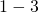2.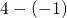3.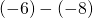4.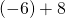5.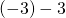6.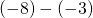7.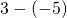8.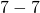9.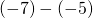10.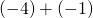11.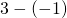12.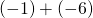13.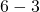14.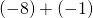15.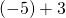16.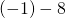17.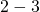18.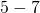19.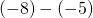20.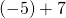21.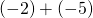22.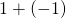23.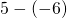24.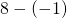25.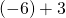26.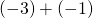27.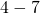28.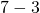29.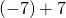30.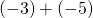For questions 31 to 44, find each product.

1.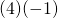2.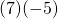3.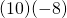4.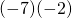5.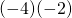6.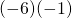7.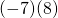8.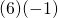9.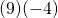10.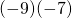11.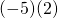12.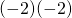13.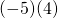14.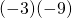For questions 45 to 58, find each quotient.

1.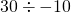2.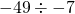3.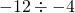4.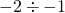5.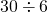6.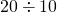7.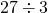8.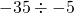9.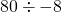10.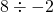11.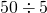12.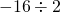13.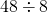14.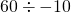2. The word "integer" is derived from the Latin word integer, which means "whole." Integers are written without using a fractional component. Examples are 2, 3, 1042, 28, 0, −42, −2. Numbers that are fractional—such as, 0.33, and 1.42—are not integers.